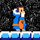cancel
Showing results for
Did you mean:Frequent Visitor

## Using 2 tables with R

I am using R script within the data transform. I know to refence to refence the curret table, you use "dataset". If there is a way, how would I refence a second data table that I have loaded in powerbi already?

ie I have a live data table that I am working with and the second table that I need to refence is a updating master name list. So if a name does not show up on the table I am working with then my code notes the name has a zero for my data or if the person is no longer around they don't show up in the report.

1 ACCEPTED SOLUTIONSuper User

Hi. I'm not sure I would create a complex transformation and etl with R or Python but yes you can use more than 1 table. You just need to take a look at PowerQuery code to modify the parameters. You can activate "Formula Bar" to check the code. That might help next time. Let's check how it works by default taking one "dataset"

``R.Execute( #"Previous Step", [dataset=#"Previous Step"] )``

If you want to use another frame there, you can just specify it in the powerquery line like:

``R.Execute(#"Previous Step", [dataset=#"Previous Step", dataset2=#"Other Step", dataset3=#"Other Table"])``

That way the script you will write latter know more than one frame. You will be able to combine in any way you want. I'm not an R developer, but here you have an example:

``````df_A <- dataset
df_B <- dataset2
df_C <- dataset3
df_temp <- rbind(df_C, df_B, df_A)
output <- .....``````

I hope that helps,

If this post helps, then please consider Accept it as the solution to help the other members find it more quickly.

Happy to help!Super User

Hi. I'm not sure I would create a complex transformation and etl with R or Python but yes you can use more than 1 table. You just need to take a look at PowerQuery code to modify the parameters. You can activate "Formula Bar" to check the code. That might help next time. Let's check how it works by default taking one "dataset"

``R.Execute( #"Previous Step", [dataset=#"Previous Step"] )``

If you want to use another frame there, you can just specify it in the powerquery line like:

``R.Execute(#"Previous Step", [dataset=#"Previous Step", dataset2=#"Other Step", dataset3=#"Other Table"])``

That way the script you will write latter know more than one frame. You will be able to combine in any way you want. I'm not an R developer, but here you have an example:

``````df_A <- dataset
df_B <- dataset2
df_C <- dataset3
df_temp <- rbind(df_C, df_B, df_A)
output <- .....``````

I hope that helps,

If this post helps, then please consider Accept it as the solution to help the other members find it more quickly.

Happy to help!